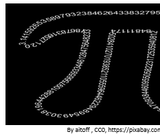# 4 Results

View
Selected filters:
• Exploring Real NumbersConditions of Use:
Remix and Share
Rating

In this seminar you will learn about the absolute value of numbers. You will learn how taking the absolute value affects both positive and negative numbers. You will use the techniques learned in this seminar to verify solutions to various other types of problems involving absolute value as you move forward. When looking at absolute value, you will identify how it can change a solution and the compare the difference that it makes in equations when there are multiple negative signs.StandardsCC.2.1.HS.F.2Apply properties of rational and irrational numbers to solve real world or mathematical problemsLearning TargetI can find the absolute value of a given number or numbers.Habits of MindPersistingCritical Thinking SkillAnalyze/evaluateAcademic/Concept VocabularyAbsolute valueNegativeNumber linePositive

Subject:
Mathematics
Algebra
Material Type:
Lesson Plan
Author:
Tracy Rains
03/19/2018Conditions of Use:
Remix and Share
Rating

In this project, you will explore a real-world problem, and then work through a series of steps to analyze that problem, research ways the problem could be solved, then propose a possible solution to that problem. Often, there are no specific right or wrong solutions, but sometimes one particular solution may be better than others. The key is making sure you fully understand the problem, have researched some possible solutions, and have proposed the solution that you can support with information / evidence.Begin by reading the problem statement in Step 1. Take the time to review all the information provided in the statement, including exploring the websites, videos and / or articles that are linked. Then work on steps 2 through 8 to complete this problem-based learning experience.This project focuses on the following:Habits of Mind: Thinking about thinking (metacognition)Critical Thinking Skills: Analyze/evaluate

Subject:
Mathematics
Algebra
Material Type:
Lesson Plan
Author:
Tracy Rains
03/27/2018Conditions of Use:
Remix and Share
Rating

In this seminar you will explore different types of numbers in math. You will learn to classify numbers into the categories of natural numbers, whole numbers, integers, rational numbers, irrational numbers, and real numbers. You will see how some numbers can be classified in a few of these categories. Some important things to consider will be whether or not a decimal number terminates or repeats.StandardsCC.2.1.HS.F.2Apply properties of rational and irrational numbers to solve real world or mathematical problems.

Subject:
Algebra
Material Type:
Lesson Plan
Author:
Tracy Rains
03/27/2018Conditions of Use:
Remix and Share
Rating

In this seminar you will explore square roots and see how they are used throughout mathematics.  You will see how “squaring” a number and “taking the square root” relate to each other. Also presented will be how to estimate square roots when given a number. You will have plenty of opportunity to try practice problems, review, and even create a short presentation. You will apply techniques you have learned involving estimating. You will use the techniques learned in this seminar to solve problems involving square roots moving forward and ultimately be able to verify solutions. When working with square roots, you will identify the patterns in perfect square numbers, as well as in techniques such as squaring and cubing numbers.  By the end, you should have a better understanding of what a square root is and how to handle expressions that involve square roots.StandardsCC.2.1.HS.F.1Apply and extend the properties of exponents to solve problems with rational exponents.CC.2.2.HS.D.6Extend the knowledge of rational functions to rewrite in equivalent forms.

Subject:
Mathematics
Algebra
Material Type:
Lesson Plan
Author:
Tracy Rains